# SAT Math Multiple Choice Question 906: Answer and Explanation

### Test Information

Question: 906

6.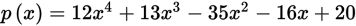Which of the following is a factor of the polynomial given above?

• A.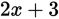• B.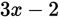• C.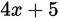• D.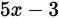Explanation:

B

Difficulty: Hard

Category: Passport to Advanced Math / Exponents

Strategic Advice: You will not be expected to factor a fourth-degree polynomial like the one in this question on Test Day, so there must be another way to approach the solution. This is a long polynomial, so think long division.

Getting to the Answer: Factors of polynomials must divide evenly into the polynomial, which means there is no remainder. In polynomial long division, the only time there is no remainder is when the constant in the factor divides evenly into the constant in the polynomial. This means you can eliminate A and D (because 3 does not divide evenly into 20). To decide between (B) and C, use long division. Start with (B):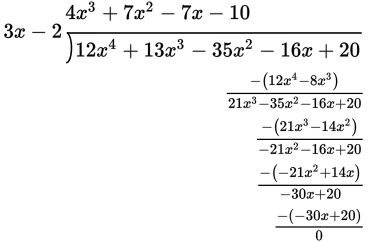When p(x) is divided by 3x - 2, the remainder is 0, so 3x - 2 is a factor of the polynomial. This means (B) is correct.